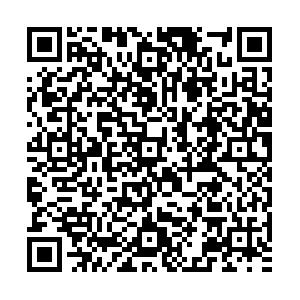# Band head spin determination of triaxial superdeformed bands in 163,164,165Lu through two-parameter formulae

• The two-parameter formulae, i.e. the nuclear softness formula and the power index formula, have been used to obtain the band head spin (I0) of the triaxial superdeformed (SD) bands in 163Lu(1, 2, 3, 4), 164Lu(1, 2, 3) and 165Lu(1, 2, 3), in the A~160 mass region. The least squares fitting approach is used. The values of the root mean square (RMS) deviation among the computed and the measured experimental transition energies are obtained by calculating the model parameters. Whenever accurate spins are available, superb agreement is shown between the determined and the measured experimental transition energies. In comparison to the power index formula, the values of band head spin (I0) of the triaxial SD bands in 163Lu(1, 2, 3, 4), 164Lu(1, 2, 3) and 165Lu(1, 2, 3) obtained by the nuclear softness formula are closer to the experimental data. The lowest RMS deviation is also achieved by the nuclear softness formula. Hence, the nuclear softness formula works well for obtaining the band head spin (I0) for the triaxial SD bands in 163Lu(1, 2, 3, 4), 164Lu(1, 2, 3) and 165Lu(1, 2, 3) in the A~160 mass region. The dynamic moment of inertia against is also studied.
PCAS:
••Get Citation
Honey Sharma and H. M. Mittal. Band head spin determination of triaxial superdeformed bands in 163,164,165Lu through two-parameter formulae[J]. Chinese Physics C, 2018, 42(11): 114102. doi: 10.1088/1674-1137/42/11/114102
Honey Sharma and H. M. Mittal. Band head spin determination of triaxial superdeformed bands in 163,164,165Lu through two-parameter formulae[J]. Chinese Physics C, 2018, 42(11): 114102.Milestone
Revised: 2018-08-02
Article Metric

Article Views(806)
Cited by(0)
Policy on re-use
To reuse of subscription content published by CPC, the users need to request permission from CPC, unless the content was published under an Open Access license which automatically permits that type of reuse.
###### 通讯作者: 陈斌, bchen63@163.com
• 1.

沈阳化工大学材料科学与工程学院 沈阳 110142

Title:
Email:

## Band head spin determination of triaxial superdeformed bands in 163,164,165Lu through two-parameter formulae

• 1. Dr. B. R. Ambedkar National Institute of Technology, Jalandhar, 144011, India

Abstract: The two-parameter formulae, i.e. the nuclear softness formula and the power index formula, have been used to obtain the band head spin (I0) of the triaxial superdeformed (SD) bands in 163Lu(1, 2, 3, 4), 164Lu(1, 2, 3) and 165Lu(1, 2, 3), in the A~160 mass region. The least squares fitting approach is used. The values of the root mean square (RMS) deviation among the computed and the measured experimental transition energies are obtained by calculating the model parameters. Whenever accurate spins are available, superb agreement is shown between the determined and the measured experimental transition energies. In comparison to the power index formula, the values of band head spin (I0) of the triaxial SD bands in 163Lu(1, 2, 3, 4), 164Lu(1, 2, 3) and 165Lu(1, 2, 3) obtained by the nuclear softness formula are closer to the experimental data. The lowest RMS deviation is also achieved by the nuclear softness formula. Hence, the nuclear softness formula works well for obtaining the band head spin (I0) for the triaxial SD bands in 163Lu(1, 2, 3, 4), 164Lu(1, 2, 3) and 165Lu(1, 2, 3) in the A~160 mass region. The dynamic moment of inertia against is also studied.

### HTML

Reference (37)
PDF查看关注分享

Top

### 目录/DownLoad:  Full-Size Img  PowerPoint